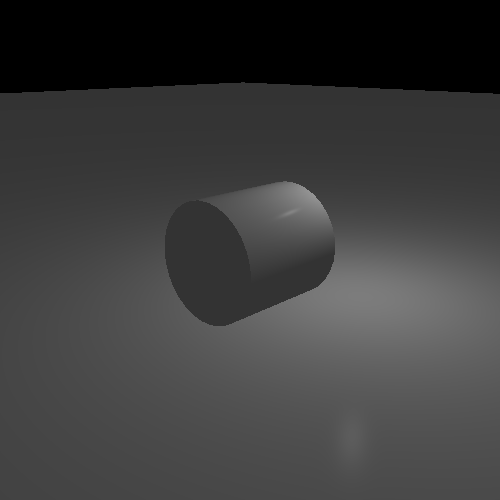## Gaussian

Phong and Blinn are nice toy heuristics that take relatively little computational power. But if you're truly serious about computing specular highlights, you need a model that actually models microfacets.

Real microfacet models are primarily based on the answer to the question What proportion of the microfacets of this surface are oriented in such a way as to specularly reflect light towards the viewer? The greater the proportion of properly oriented microfacets, the stronger the reflected light. This question is ultimately one of statistics.

Thus it makes sense to model this as a probability distribution. We know that the average microfacet orientation is the surface normal. So it's just a matter of developing a probability distribution function that says what portion of the surface's microfacets are oriented to provide specular reflections given the light direction and the view direction.

In statistics, the very first place you go for modelling anything with a probability distribution is to the normal distribtuion or Gaussian distribution. It may not be the correct distribution that physically models what the microfacet distribution of a surface looks like, but it's usually a good starting point.

The Gaussian distribution is the classic bell-shaped curve distribution. The mathematical function for computing the probability density of the Gaussian distribution at a particular point X is:

Equation 11.5. Gaussian Distribution Function

$f\left(x\right)=\frac{1}{\sqrt{2\pi \sigma }}{e}^{-\frac{{\left(x-\mu \right)}^{2}}{2{\sigma }^{2}}}$

Figure 11.14. Gaussian Probability Distribution CurvesThis represents the percentage of the items in the distribution that satisfy the property that the X in the distribution is trying to model. The e in this equation is a common mathematical constant, equivalent to ~2.718. The value of μ is the average. So the absolute value of X is not important; what matters is how far X is from the average.

The value σ2 is the variance of the Gaussian distribution. Without getting too technical, the larger this value becomes, the flatter and wider the distribution is. The variance specifies how far from the average you can get to achieve a certain probability density. The area of the distribution that is positive and negative σ away from the average takes up ~68% of the possible values. The area that is 2σ away represents ~95% of the possible values.

We know what the average is for us: the surface normal. We can incorporate what we learned from Blinn, by measuring the distance from perfect reflection by comparing the surface normal to the half-angle vector. Thus, the X values represents the angle between the surface normal and half-angle vector. The value μ, the average, is zero.

The equation we will be using for modelling the microfacet distribution with a Gaussian distribution is a slightly simplified form of the Gaussian distribution equation.

Equation 11.6. Gaussian Specular Term

$\begin{array}{l}\alpha :\text{Angle between H and N}\\ \mathrm{Gaussian Term}={e}^{-{\left(\frac{\alpha }{m}\right)}^{2}}\end{array}$

This replaces our Phong and Blinn terms in our specular lighting equation and gives us the Gaussian specular model. The value m ranges from (0, 1], with larger values representing an increasingly rougher surface. Technically, you can use values larger than 1, but the results begin looking increasingly less useful. A value of 1 is plenty rough enough for specular reflection; properly modelling extremely rough surfaces requires additional computations besides determining the distribution of microfacets.

The Gaussian Specular Lighting tutorial shows an implementation of Gaussian specular. It allows a comparison between Phong, Blinn, and Gaussian. It controls the same as the previous tutorial, with the H key switching between the three specular computations, and the Shift+H switching between diffuse+specular and specular only.

Here is the fragment shader for doing Gaussian lighting.

Example 11.3. Gaussian Lighting Shader

vec3 lightDir = vec3(0.0);
float atten = CalcAttenuation(cameraSpacePosition, lightDir);
vec4 attenIntensity = atten * lightIntensity;

vec3 surfaceNormal = normalize(vertexNormal);
float cosAngIncidence = dot(surfaceNormal, lightDir);
cosAngIncidence = clamp(cosAngIncidence, 0, 1);

vec3 viewDirection = normalize(-cameraSpacePosition);

vec3 halfAngle = normalize(lightDir + viewDirection);
float angleNormalHalf = acos(dot(halfAngle, surfaceNormal));
float exponent = angleNormalHalf / shininessFactor;
exponent = -(exponent * exponent);
float gaussianTerm = exp(exponent);

gaussianTerm = cosAngIncidence != 0.0 ? gaussianTerm : 0.0;

outputColor = (diffuseColor * attenIntensity * cosAngIncidence) +
(specularColor * attenIntensity * gaussianTerm) +
(diffuseColor * ambientIntensity);

Computing the angle between the half-angle vector and the surface normal requires the use of the acos function. We use the dot-product to compute the cosine of the angle, so we need a function to undo the cosine operation. The arc cosine or inverse cosine function takes the result of a cosine and returns the angle that produced that value.

To do the exponentiation, we use the exp function. This function raises the constant e to the power of the argument. Everything else proceeds as expected.

### What Gaussian Offers

If you play around with the controls, you can see how much the Gaussian distribution offers over Phong and Blinn. For example, set the Gaussian smoothness value to 0.05.

Figure 11.15. Gaussian with Sharp HighlightIt requires very large exponents, well in excess of 100, to match the small size and focus of that specular highlight with Phong or Blinn. It takes even larger exponents to match the Gaussian value of 0.02.

Otherwise the differences between Gaussian and Blinn are fairly subtle. For rough surfaces, there is little substantive difference. But Gaussian tends to have a sharper, more distinct highlight for shiny surfaces.

Fork me on GitHub